# Logistic Regression

###### Introduction

Logistic regression is a parametric algorithm for solving classification problems whereby the label,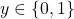. Logistic regression and perceptron are similar whereby a hyperplane is defined to divide the feature space into regions labeled according to the classification. The hyperplane is defined by the inner product between parameters the and the input vectors (summation of the weighted inputs),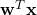. Then, the resultant of the inner product is passed to a function that ensures the predicted value to be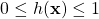via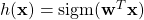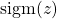refers to the sigmoid function, also known as logistic function which is defined as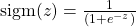Figure 1 shows the graph of the sigmoid function. The sigmoid function has an S-shaped curve, which maps its input to a value within the range of 0 and 1. Thus, logistic regression has a probabilistic connotation. The curve has two horizontal asymptotes, one end is approaching 1 and the other end is approaching 0. Due to this property, the sigmoid function is differentiable at every point.

As mentioned above, a logistic regression model outputs values within the range of 0 and 1. If we threshold the output at 0.5, a classification rule can be defined as follows: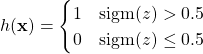Figure 2 shows the output probabilities of 50 simulated inputs to the sigmoid function. The input values vary from -17 to +22 and their predicted values are indicated by the red circles. Using a threshold value of 0.5, the classification of the data is indicated by the green and blue markers. As can be seen in the figure, output probabilities above the threshold value are classified as 1. Otherwise, they are classified as 0.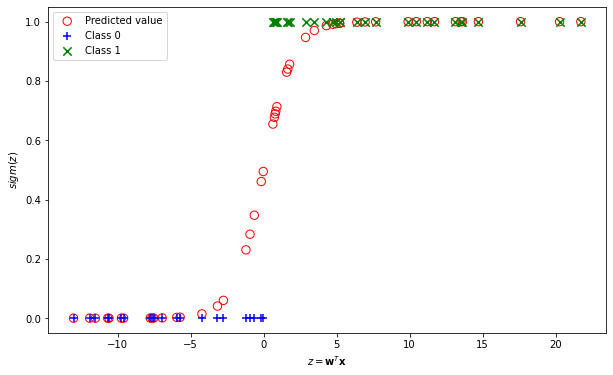Figure 2. The output probabilities of the sigmoid function and their classification using a threshold value of 0.5.

Numerical Example

Consider the logistic regression model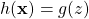where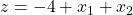. Predict the labels of the following inputs.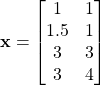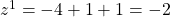,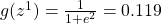,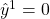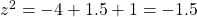,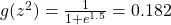,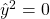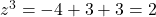,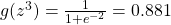,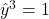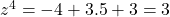,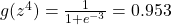,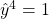###### Multiclass Classification Setting

So far, we have seen logistic regression for binary classification. Real-world problems may involve more than two classes. For multiclass classification, we need to train multiple logistic regression models, one for each of the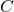classes. For example, consider a classification problem as illustrated in Figure 3 (top). The data points belong to three classes, RED, BLUE and GREEN. We build three logistic regression models for each of the three classes. First, we treat RED instances as class 1, and all the other instances as class 0. Then, we build the logistic regression model to distinguish RED instances from the rest. Next, we treat BLUE instances as class 1 and all the other instances as class 0, and build the logistic regression model to distinguish BLUE instances from the rest. We perform the same method for GREEN instances. Eventually, we have three logistic regression models as shown in Figure 3 (bottom).

Given a test point,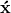, all models perform the prediction on the test point and producepredicted values.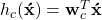Then, the predicted values are normalized using the softmax function. The softmax function turns the predicted values into probabilities. Each predicted value will be in the range of 0 and 1 and the sum of the values equal to 1.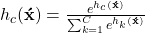The test point is assigned to the class with the largest probability.

###### Parameter Estimation

Now, how do we estimate the parameters of the logistic regression? We will discuss the estimation of the parameters for two-class logistic regression to simplify the explanation. The loss function of logistic regression is the negative log-likelihood or cross entropy as follows: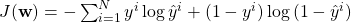where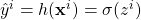,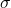denotes the sigmoid function and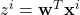We take the derivative of the loss function with respect to the parameters and set to zero. Using chain rule, the derivate of the loss function is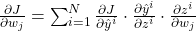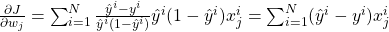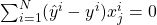However, the maximum likelihood estimation of the parameters are not in closed form. Thus, the equation needs to be solved using optimization algorithm such as gradient descent, Newton’s method etc. Using gradient descent, the parameters are estimated as follows: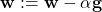where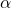is the learning rate and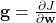is the gradient or the derivative of the loss function with respect to the parameters.

Using Newton’s method, the parameters are estimated as follows: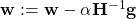where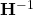is the Hessian matrix or the second-order partial derivatives of the loss function with respect to the parameters.